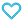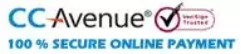# NCERT Solutions Mathematics Class 11

175.00

Discount offer on this book in a bundle, click to view

MTG NCERT Solutions Mathematics enhances the problem-solving skills of the students. The book provides complete solutions to all types of questions asked in NCERT Mathematics Textbook.

943 in stock

Qty:Add to WishlistNCERT Books are the key book for every class. Analysed and reviewed by teachers Pan India, these books ensure a smooth and easy understanding in stepwise manner. The reading of these books is a must for every student. This become more important for the students of higher class like 11 and 12 where competitive exams strictly follow NCERT books. To ensure that students get the right answer as per the requirement MTG has designed NCERT Solution Books. The best strategy to use these Solution book is to solve the NCERT questions first themselves and then review their answers from Solution book. Having a significant recognition in the sphere of Study materials for XI and XII science stream, the MTG experts have provided best NCERT Solutions in these books. These aid students with their homework, while preparing for exams and while learning.

Table of Content :
• 1. Sets
• 2. Relations and Functions
• 3. Trigonometric Functions
• 4. Principle of Mathematical Induction
• 5. Complex Numbers and Quadratic Equations
• 6. Linear Inequalities
• 7. Permutations and Combinations
• 8. Binomial Theorem
• 9. Sequences and Series
• 10. Straight Lines
• 11. Conic Sections
• 12. Introduction to Three Dimensional Geometry
• 13. Limits and Derivatives
• 14. Mathematical Reasoning
• 15. Statistics
• 16. Probability
ISBN11 9789389461473 MTG Editorial Board 2019-20 168 Class 11 School Books Mathematics 210gm

## Reviews

There are no reviews yet.